International
Tables for
Crystallography
Volume G
Definition and exchange of crystallographic data
Edited by S. R. Hall and B. McMahon

International Tables for Crystallography (2006). Vol. G, ch. 2.3, pp. 42-43

Section 2.3.5. imgCIF encodings

H. J. Bernsteina* and A. P. Hammersleyb

aDepartment of Mathematics and Computer Science, Kramer Science Center, Dowling College, Idle Hour Blvd, Oakdale, NY 11769, USA, and bESRF/EMBL Grenoble, 6 rue Jules Horowitz, France
Correspondence e-mail:  yaya@bernstein-plus-sons.com

2.3.5. imgCIF encodings

| top | pdf |

For an imgCIF, there are several alternative encodings for binary image data as ASCII text. Each binary image may use a different encoding in the same imgCIF data set or even in the same data block. The choice of encoding is specified in the `Content-Transfer-Encoding' MIME header.

If the Transfer Encoding is X-BASE8, X-BASE10 or X-BASE16, the data are presented as octal, decimal or hexadecimal data, respectively, organized into lines or words. Each word is created by composing octets of data in fixed groups of 2, 3, 4, 6 or 8 octets, either in the order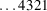(`big-endian') or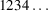(`little-endian'). If there are fewer than the specified number of octets to fill the last word, then the missing octets are presented as `==' for each missing octet. Exactly two equal signs are used for each missing octet even for octal and decimal encoding. The format of lines is

rnd xxxxxx xxxxxx xxxxxx

where r is H, O or D for hexadecimal, octal or decimal, n is the number of octets per word, and d is `<' or `>' for the `' and `' octet orderings, respectively. The `==' padding for the last word should be on the appropriate side to correspond to the missing octets, e.g.or

H3> FF0700 00====

For these hexadecimal, octal and decimal formats only, comments beginning with `#' are permitted to improve readability.

BASE64 encoding follows MIME conventions. Octets are in groups of three, c1, c2, c3. The resulting 24 bits are reorganized in the following way (where we use the C operators,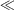& and | to denote, respectively, a right shift, a left shift, bit-wise intersection and bit-wise union). Four six-bit quantities are specified, starting with the high-order six bits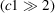of the first octet, then the low-order two bits of the first octet followed by the high-order four bits of the second octet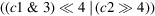, then the bottom four bits of the second octet followed by the high-order two bits of the last octet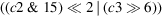, then the bottom six bits of the last octet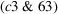. Each of these four quantities is translated into an ASCII character using the mappingShort groups of octets are padded on the right with one `=' if c3 is missing, and with `==' if both c2 and c3 are missing.

QUOTED-PRINTABLE encoding also follows MIME conventions, copying octets without translation if their ASCII values are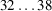, 42,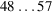,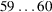, 62,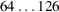and the octet is not a ` ;' in column 1. All other characters are translated to `=nn, where nn is the hexadecimal encoding of the octet. All lines are `wrapped' with a terminating `=' (i.e. the MIME conventions for an implicit line terminator are never used).